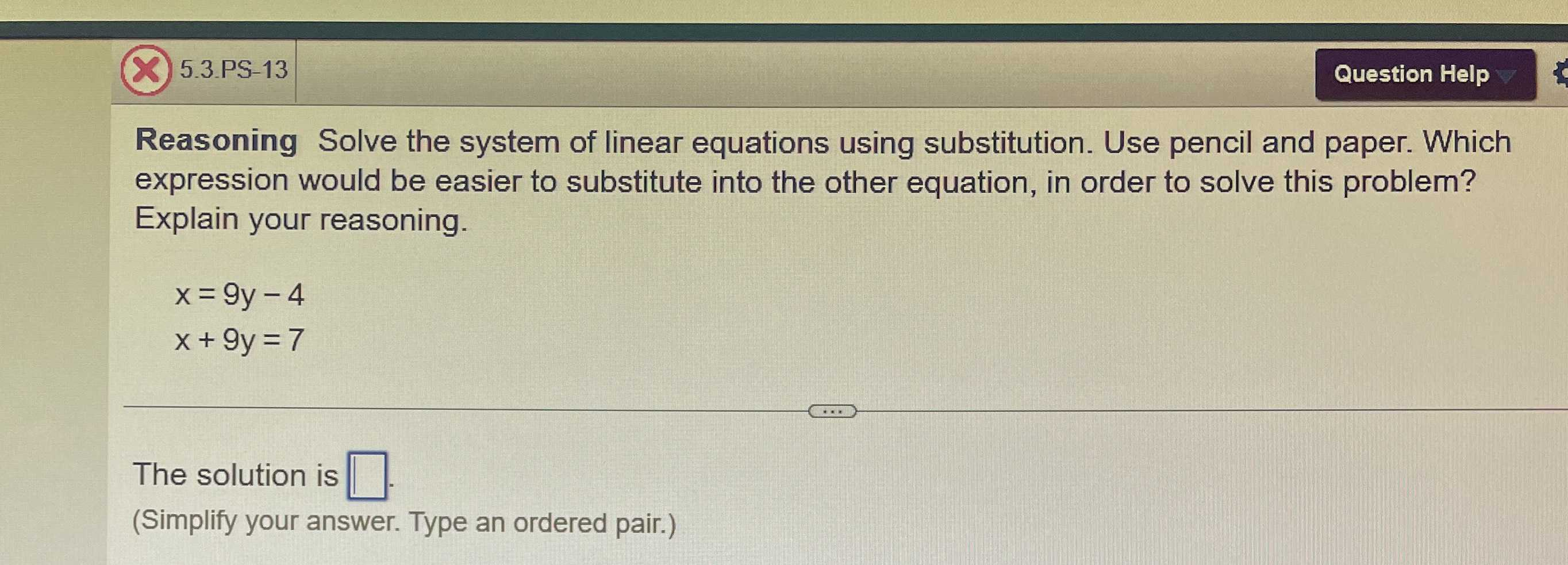### ¿Todavía tienes preguntas de matemáticas?

Pregunte a nuestros tutores expertos
Algebra
Pregunta5.3.PS-13 Reasoning Solve the system of linear equations using substitution. Use pencil and paper. Which expression would be easier to substitute into the other equation, in order to solve this problem? Explain your reasoning.

$$x = 9 y - 4$$

$$x + 9 y = 7$$

The solution is $$\square$$ .

x= 9y-4

x+9y=7                         ..............(2)

substituting value of x in equation  (2) because it will be easy to calculate

9y-4+9y=7

18y=11

y=$$\frac{11}{18}$$

x=9($$\frac{11}{18}$$)+4=$$\frac{171}{18}$$

Solución
View full explanation on CameraMath App.